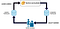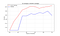# Introduction

We are currently immersed in a large quantity of data and information which we can use to create efficient models of machine learning. The biggest drawback we found when we are working with supervised models is that we don’t have enough tagged data. Data tagging involves a great effort in time and cost.

## How to use this technique?

Let’s start with an example, if we only had the capacity in time and effort to tag only 500 data in order to improve our models, this strategy will allow us to take those 500 data to optimize our model with a lower cost, the following graphic shows the process described before.Pipeline Active Learning

# Pool-Based Sampling

In this stage we assume that we have already some tagged data, (a subset L), and another set of unlabeled data U. In each iteration, a subsample, which belongs to U, is selected to be tagged for a human annotator.

• Variance Reduction: This strategy selects a subset of unlabeled data in order to reduce the variance of the final model. This way we are indirectly minimising the error of generalization of the trained model. However, this strategy could be very computational expensive.
• Expected Error Reduction: Selecting a subset of data to posteriori decrease generalization error of the model, that would reduce the false positive rate.
• Query-By-Committee (QBC): This strategy trains a set of models with the data tagged. These trained model will predict new unlabeled data. At the end the data that differ the most in the prediction will be sent to tag.

# Pipeline

In this dummy example, I will go to use iris dataset to do validation of how Active Learning helps to improve models. I will use a library called modAL  to do an example, using uncertainty sampling strategy and later I will train another model with random sampling strategy.

`import pandas as pdimport numpy as npimport matplotlib.pyplot as pltfrom sklearn.datasets import load_iris# load datasetiris = load_iris()X_raw = iris['data']y_raw = iris['target']`
`# split datasetn_labeled_examples = X_raw.shapetraining_indices = np.random.randint(low=0, high=n_labeled_examples + 1, size=5)X_train = X_raw[training_indices]y_train = y_raw[training_indices]# Isolate the non-training examples we'll be querying.X_pool = np.delete(X_raw, training_indices, axis=0)y_pool = np.delete(y_raw, training_indices, axis=0)`
`learner = ActiveLearner(estimator=RandomForestClassifier(),                        query_strategy=uncertainty_sampling,                        X_training=X_train, y_training=y_train)unqueried_score = learner.score(X_raw, y_raw)`
`clf, list_history = loop(learner, X_pool, y_pool, performance_history=[unqueried_score])`
`def random_sampling(classifier, X_pool):    query_idx = np.random.choice(range(X_pool.shape))    return query_idx, X_pool[query_idx]# create a random learner object with the strategies created before.learner_random = ActiveLearner(estimator=RandomForestClassifier(),                               query_strategy=random_sampling,                               X_training=X_train, y_training=y_train)clf, list_history_random = loop(learner_random, X_pool, y_pool, performance_history=[unqueried_score])`Active Learning VS Random Sample.

# Conclusions

As can be seen, using a strategy to label data more intelligently helps improve the model’s metrics with much less data.

# References

Data Scientist at Mercado Libre

## More from Elbio Zapata

Data Scientist at Mercado Libre